Chin. Phys. B, 2020, Vol. 29(10): 104204    DOI: 10.1088/1674-1056/ab9de8
 ELECTROMAGNETISM, OPTICS, ACOUSTICS, HEAT TRANSFER, CLASSICAL MECHANICS, AND FLUID DYNAMICS Prev   Next

# Dependence of interferogram phase on incident wavenumber and phase stability of Doppler asymmetric spatial heterodyne spectroscopy

Ya-Fei Zhang(张亚飞)1,2, Yu-Tao Feng(冯玉涛)1,†(), Di Fu(傅頔)1, Peng-Chong Wang(王鹏冲)1, Jian Sun(孙剑)1, Qing-Lan Bai(白清兰)1
1 Xi’an Institute of Optics and Precision Mechanics, Chinese Academy of Sciences, Xi’an 710119, China
2 University of Chinese Academy of Sciences, Beijing 100049, China
Abstract

Instrument drifts introduce additional phase errors into atmospheric wind measurement of Doppler asymmetric spatial heterodyne spectroscopy (DASH). Aiming at the phase sensitivity of DASH to instrument drifts, in this paper we calculate the optical path difference (OPD) and present an accurate formula of DASH interferogram. By controlling variables in computational ray-tracing simulations and laboratory experiments, it is indicated that initial phase is directly determined by incident wavenumber, OPD offset and field of view (FOV). Accordingly, it is indicated that retrieved phase of DASH is sensitive to slight structural change caused by instrument drift, which provides the proof of necessary-to-track and -correct phase errors from instrument drifts.

Keywords:  atmospheric wind measurement      Doppler asymmetric spatial heterodyne spectroscopy      optical path difference      interference phase
Received:  07 January 2020      Revised:  30 April 2020      Published:  05 October 2020
 PACS: 42.25.Hz (Interference) 07.60.Ly (Interferometers) 42.68.-w (Atmospheric and ocean optics)
Corresponding Authors:  Corresponding author. E-mail: fytciom@126.com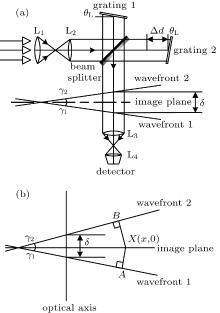Fig. 1.  (a) Schematic diagram of typical DASH interferometer, where L1 and L2 are collimating lenses. L3 and L4 are imaging lenses, gratings 1 and 2 are tilted by a Littrow angle θL, Δd is the asymmetric offset of two optical arms. Outgoing wavefronts have the same phase. (b) Schematic diagram of the calculation of OPD. Because wavefronts 1 and 2 have the same phase, the OPD at point X on image plane can be obtained by |BX| + |XA|.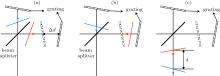Fig. 2.  Schematic diagram of wavefronts movement approximation, where red and blue lines represent wavefronts and arrows denote the direction of approximated wavefronts.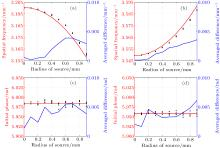Fig. 3.  FOV effects and comparisons at [(a) and (c)] 15873 cm−1 and [(b) and (d)] 15803 cm−1. Red lines represent results calculated from Eq. (5). Black dots denote simulation results. Blue lines indicate averaged difference between calculation and simulation results. Averaged difference is obtained by averaging the absolute values of all differences.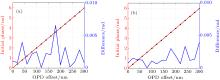Fig. 4.  Phase comparisons of OPD offset effect (a) at 15873 cm−1 and (b) at 15803 cm−1. Red lines represent results calculatedfrom Eq. (5). Black triangles represent simulation results. Blue lines indicate averaged difference between calculation and simulation results.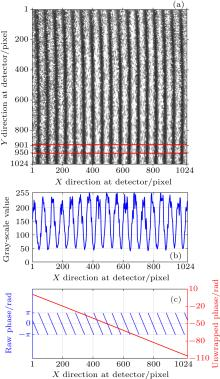Fig. 5.  (a) Averaged interferogram from five recorded pictures. (b) Analysis signal generated from the selected area shown in panel (a). (c) Schematic diagram of inversion phase from analysis signal shown in panel (b). Blue points indicate the retrieved raw phase and red points reptrsent the unwrapped phase.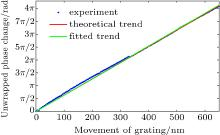Fig. 6.  Phase variations at 512nd pixel when grating on the linear stage is gradually moved.